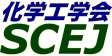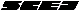## \$B9V1i%W%m%0%i%`!J2q>l!&F|DxJL!K(B

### T\$B2q>l(B \$BBh(B1\$BF|(B

\$B:G=*99?7F|;~!'(B2012-08-20 15:02:17
\$B9V1i(B
\$B;~9o(B
\$B9V1i(B
\$BHV9f(B
\$B9V1iBjL\!?H/I=\$B%-!<%o!<%I(B\$BJ,N`(B
\$BHV9f(B
\$B\$BHV9f(B
\$B%7%s%]%8%&%`(B \$B!cG.9)3X%7%s%]%8%&%`!d(B
(9:00\$B!A(B10:00)\$B!!(B(\$B:BD9(B \$B;3K\(B \$B9d(B)
9:00\$B!A(B 9:20T101EV\$B\$rG^BN\$H\$7\$?EENO6!5k%7%9%F%`\$N4pAC8&5f(B
(\$B2,;38)Bg1!(B) \$B!{(B(\$B3X(B)\$BLZ2<(B \$BM5G7(B \$B!&(B (\$B3X(B)\$B8E@%(B \$BE590(B \$B!&(B \$BK~K\(B \$BM4B@(B \$B!&(B (\$B@5(B)\$BCf@n(B \$BFsI'(B
electric vehicle
photovoltaics
smart grid
S-3369
9:20\$B!A(B 9:40T102HEX\$B%b%G%k\$rMQ\$\$\$?(BPV\$BEENO\$NM-8z3hMQK!(B
(\$B2,;38)Bg(B) \$B!{(B(\$B3X(B)\$B8E@%(B \$BE590(B \$B!&(B (\$B3X(B)\$BLZ2<(B \$BM5G7(B \$B!&(B (\$B3X(B)\$BK~K\(B \$BM4B@(B \$B!&(B (\$B@5(B)\$BCf@n(B \$BFsI'(B
Photovoltaic power generation
Electric Vehicle
Energy System
S-3591
9:40\$B!A(B 10:00T103\$B??Dm(BSMART\$B9)>l\$K\$*\$1\$k:F@82DG=%(%M%k%.!<\$N3hMQ%7%9%F%`(B
(\$B2,;38)Bg(B) \$B!{(B(\$B3X(B)\$B;3K\(B \$B??Bg(B \$B!&(B (\$B@5(B)\$BCf@n(B \$BFsI'(B
Photovoltaic power generation
Electric forklift truck
Energy System
S-3620
(10:00\$B!A(B11:00)\$B!!(B(\$B:BD9(B \$BHDC+(B \$B5A5*(B)
10:00\$B!A(B 10:20T104\$B5[Ce<0>x5\$@8@.%R!<%H%]%s%W\$K\$*\$1\$k@8@.>x5\$NL\$N2~A1J}K!\$K4X\$9\$k8!F\$(B
(\$B6eBg1!9)(B) \$B!{(B(\$B3X(B)\$B4d4V(B \$B2BJf(B \$B!&(B (\$B3X(B)\$BEDCf(B \$BM\$ \$B!&(B (\$B3X(B)\$BCfEg(B \$B?tLp(B \$B!&(B Xue Bing \$B!&(B (\$B6eBg9)(B) (\$B@5(B)Agung Tri Wijayanta \$B!&(B (\$B@5(B)\$BCfA>(B \$B9@0l(B \$B!&(B (\$B@5(B)\$B?<0f(B \$B=a(B
heat pump
steam generation
S-3479
10:20\$B!A(B 10:40T105\$B5[Ce<0>x5\$@8@.%R!<%H%]%s%W\$N%5%\$%/%k
(\$B6eBg1!9)(B) \$B!{(B(\$B3X(B)\$BEDCf(B \$BM\$ \$B!&(B (\$B3X(B)\$B4d4V(B \$B2BJf(B \$B!&(B (\$B3X(B)\$BCfEg(B \$B?tLp(B \$B!&(B Xue Bing \$B!&(B (\$B6eBg9)(B) (\$B@5(B)Agung Triwijayanta \$B!&(B (\$B@5(B)\$BCfA>(B \$B9@0l(B \$B!&(B (\$B@5(B)\$B?<0f(B \$B=a(B
heat pump
steam generation
S-3485
10:40\$B!A(B 11:00T106\$BI9E@2
(\$BH,9)Bg(B) (\$B@5(B)\$BLnED(B \$B1QI'(B \$B!&(B \$B!{(B(\$B3X(B)\$BJ?;3(B \$BC#Li(B
Absorption Refrigerator
Cold Heat
New Working Fluid
S-3835
(11:00\$B!A(B12:00)\$B!!(B(\$B:BD9(B \$B4dK\(B \$B8w@8(B)
11:00\$B!A(B 11:20T107DFR\$BG.8r495!7?9b5!G=>=@OAuCV\$N3+H/(B
(DFR\$B5;8&(B) \$B!{(B(\$B@5(B)\$B@iMU(B \$BM[0l(B \$B!&(B (\$B0l4X9b@l(B) (\$B@5(B)\$BFs3,F2(B \$BK~(B \$B!&(B (\$B4d<(B \$B!&(B (DFR\$B5;8&(B) \$B9b66(B \$B7r;J(B
Crystallization
DFR Heat Exchanger
Polymorphism Control
S-3227
11:20\$B!A(B 11:40T108DFR\$BG.8r495!\$NEAG.FC@-\$HL)JD7?Nd5QAuCV\$N3+H/(B
(DFR\$B5;8&(B) \$B!{(B(\$B@5(B)\$B@iMU(B \$BM[0l(B \$B!&(B (\$B<e(B \$BMN(B \$B!&(B (\$BH,8M9)Bg(B) (\$B@5(B)\$BLnED(B \$B1QI'(B
DFR Heat Exchanger
Cooling Apparatus
Energy Saving
S-3236
11:40\$B!A(B 12:00T109\$B9b29%o!<%/:`\$rBP>]\$H\$7\$?Hy:Y%_%9%HNd5Q\$NNd5QFC@-\$*\$h\$S@\?(G.Dq93\$r9MN8\$7\$?G.EAF35sF02r@O(B
(\$B6eBg1!9)(B) \$B!{(B(\$B3X(B)\$B5HLn(B \$BfF(B \$B!&(B (\$B@5(B)\$B;3K\(B \$B9d(B \$B!&(B (\$B@5(B)\$B?<0f(B \$B=a(B
fine mist cooling
contact heat resistance
high heat flux
S-376
(13:00\$B!A(B14:00)\$B!!(B(\$B:BD9(B \$B>>2<(B \$BMN2p(B)
13:00\$B!A(B 13:40T113[\$B>7BT9V1i(B]\$B<+M3I=LL\$rH<\$&:.AjN.\$N9b@:EY2r@O(B
(\$B=;M'6bB0(B) \$B!{(B(\$B@5(B)\$B9bC+(B \$B9,;J(B
VOF
Level Set
CFD
S-3140
13:40\$B!A(B 14:00T115[\$BM%=(O@J8>^(B]Relationship between Applied Static Magnetic Field Strength and Thermal Conductivity Values of Molten Materials Measured Using the EML Technique
(\$BElKLBg1!9)(B) (\$B3X(B)Baba Yuya \$B!&(B \$B!{(B(\$B@5(B)Sugioka Ken-ichi \$B!&(B (\$B@5(B)Kubo Masaki \$B!&(B (\$B@5(B)Tsukada Takao \$B!&(B (\$BElKLBgB?858&(B) Sugie Kazutoshi \$B!&(B Kobatake Hidekazu \$B!&(B Fukuyama Hiroyuki
Static magnetic field
Electromagnetically levitated droplet
Thermal conductivity
S-334
(14:00\$B!A(B15:20)\$B!!(B(\$B:BD9(B \$BCfA>(B \$B9@0l(B)
14:00\$B!A(B 14:20T116\$BD62;GH%I%C%W%i!<'>l0u2C(BCz\$BK!M;1UBPN.\$NB,Dj(B
(\$BBgJ,Bg9)(B) \$B!{(B(\$B3X(B)\$B:4F#(B \$BBg \$B!&(B (\$B3X(B)\$BF~;3(B \$BE/O:(B \$B!&(B \$B>.5\(B \$B7rB@O:(B \$B!&(B (\$B;37ABg9)(B) (\$B@5(B)\$B@V>>(B \$B@5?M(B \$B!&(B (\$BBgJ,Bg9)(B) \$Bc7F#(B \$B?80l(B \$B!&(B (\$B@5(B)\$B4dK\(B \$B8w@8(B
RMCz method
Lorentz force
Ultrasonic Velocity Profiler
S-3252
14:20\$B!A(B 14:40T117\$B2sE><'>l2<\$K\$*\$1\$k(BCz\$BK!M;1UBPN.\$N?tCM2r@OE*8&5f(B
(\$BBgJ,Bg9)(B) \$B!{(B(\$B3X(B)\$BF~;3(B \$BE/O:(B \$B!&(B (\$B3X(B)\$B>.ED(B \$B>)(B \$B!&(B (\$B;37ABg9)(B) (\$B@5(B)\$B@V>>(B \$B@5?M(B \$B!&(B (\$BBgJ,Bg9)(B) \$Bc7F#(B \$B?80l(B \$B!&(B (\$B@5(B)\$B4dK\(B \$B8w@8(B
Czochralski method
Rotational magnetic field
Lorentz force
S-3251
14:40\$B!A(B 15:00T118\$BEEN.(B-\$B<'>l0u2C(BCz\$BK!\$K\$*\$1\$k%k%D%\FbBPN.\$K4X\$9\$k8&5f(B
(\$BBgJ,Bg9)(B) \$B!{(B(\$B3X(B)\$B>.ED(B \$B>)(B \$B!&(B (\$B3X(B)\$B:4F#(B \$BBg \$B!&(B \$BN)@n(B \$BG=@.(B \$B!&(B (\$B;37ABg9)(B) (\$B@5(B)\$B@V>>(B \$B@5?M(B \$B!&(B (\$BBgJ,Bg9)(B) \$Bc7F#(B \$B?80l(B \$B!&(B (\$B@5(B)\$B4dK\(B \$B8w@8(B
EMCz method
Lorentz force
Ultrasonic Doppler method
S-3248
15:00\$B!A(B 15:20T119\$B%=%k%\%5!<%^%kK!\$K\$h\$k%P%k%/C17k>=0i@.;~\$NG.2r@O(B
(\$B;:Am8&(B) \$B!{(B(\$B@5(B)\$BA}ED(B \$BA1M:(B \$B!&(B (\$B@5(B)\$BNkLZ(B \$BL@(B \$B!&(B (\$BElKLBgB?858&(B) \$B@P9u(B \$BE0(B \$B!&(B (\$B@5(B)\$B2#;3(B \$B@i><(B
Natural Convection
Numerical Simulation
Solvothermal method
S-3364
(15:20\$B!A(B16:20)\$B!!(B(\$B:BD9(B \$Bc7F#(B \$BBYMN(B)
15:20\$B!A(B 15:40T120Numerical Study of Devolatilization Effect on CO/H2 Production from Coal Gasification
(Kyu U.) \$B!{(B(\$B3X(B)Alam Md Saiful \$B!&(B (\$B@5(B)Wijayanta Agung Tri \$B!&(B (\$B@5(B)Nakaso Koichi \$B!&(B (\$B@5(B)Fukai Jun
devolatilization
coal gasification
CO and H\$2\$
S-3375
15:40\$B!A(B 16:00T121\$B%^%\$%/%mGHHsJ?9U%W%i%:%^\$rMQ\$\$\$?;Y1gJ.L8G3>FFC@-\$K4X\$9\$k8!F\$(B
(\$B6eBg1!9)(B) \$B!{(B(\$B3X(B)\$B:#N\$(B \$BN<2p(B \$B!&(B (\$B@5(B)\$B;3K\(B \$B9d(B \$B!&(B (\$B@5(B)\$B?<0f(B \$B=a(B \$B!&(B (\$B0&CNEE5!(B) \$BEDCf(B \$BNI(B
non-equilibrium plasma
spray combustion
microwave
S-377
16:00\$B!A(B 16:20T122\$BHyJ,C:G3>F\$K\$*\$\$\$FJ70O5\$%,%9
(\$B6eBgC:%;(B) \$B!{(B(\$B@5(B)\$BLn_7(B \$BAOJ?(B \$B!&(B (\$B@5(B)\$B>>2<(B \$BMN2p(B
Pulverized coal combustion
Numerical simulation
S-3943
(16:20\$B!A(B17:20)\$B!!(B(\$B:BD9(B \$B?<0f(B \$B=a(B)
16:20\$B!A(B 16:40T123\$B3lC:\$N4%AgFC@-\$H3lC:Cf\$K4^\$^\$l\$k?e\$NJ*M}E*@-
(\$B6eBgC:%;(B) \$B!{(B(\$B@5(B)\$B>>2<(B \$BMN2p(B \$B!&(B \$B8w86(B \$BG5N\$;R(B \$B!&(B (\$B6eEE;:6H(B) (\$B6&(B)\$BBg?9(B \$B4pJ?(B \$B!&(B (\$B6eEE(B) (\$B6&(B)\$B86ED(B \$BC#O/(B \$B!&(B (\$B@n=E(B) \$B7'ED(B \$B7{I'(B
Brown coal
Drying
Physical properties
S-3961
16:40\$B!A(B 17:00T124\$B2
(\$B4tIlBg9)(B) \$B!{(B(\$B3X(B)\$B?\LV(B \$B6G(B \$B!&(B (\$BL>Bg9)(B) (\$B@5(B)\$B>.NS(B \$B?.2p(B \$B!&(B (\$B%*%*%V%f%K%F%#(B) \$BIMJU(B \$B5W(B \$B!&(B \$B_70f(B \$B@5OB(B \$B!&(B (\$B4tIlBg9)(B) (\$B@5(B)\$BHDC+(B \$B5A5*(B
Water treatment
Carbonization
Sewage drying
S-3635
17:00\$B!A(B 17:20T125\$B22A(B
(\$BElKLBg1!9)(B) \$B!{(B(\$B3X(B)\$BG\>^(B \$B90J?(B \$B!&(B (\$B@5(B)\$Bc7F#(B \$BBYMN(B \$B!&(B (\$B@5(B)\$B@DLZ(B \$B=(G7(B
assessment
botryococcus braunii
aurantiochytrium limacinum
S-3241

\$B9V1i%W%m%0%i%`(B
\$B2=3X9)3X2q(B \$BBh(B44\$B2s=)5(Bg2q(B(C) 2012 \$B8x1W
Most recent update: 2012-08-20 15:02:17
For more information contact \$B2=3X9)3X2qElKL;YIt(B \$BBh(B44\$B2s=)5(Bg2q(B \$BLd\$\$9g\$;78(B
E-mail: inquiry-44fwww3.scej.org
This page was generated byeasp 2.28; proghtml 2.28c (C)1999-2011 kawase